## Overview

Every linear optimization problem can be expressed as a set of linear inequalities and an objective function to be maximized or minimized. The inequalities are called constraints, and like all inequalities, they contain variables.

When you install the linear optimization add on, you'll be able to populate your spreadsheet with variables and constraints, click Solve, and see the solution to your problem. This documentation shows you how.

Before you can solve linear optimization problems from a spreadsheet, you need to install the add-on that makes it possible. On that page, just click INSTALL to install the add-on.

Once you install the add-on and grant it the necessary permissions, you'll be placed into an empty spreadsheet ready for your variables and constraints.

## A simple example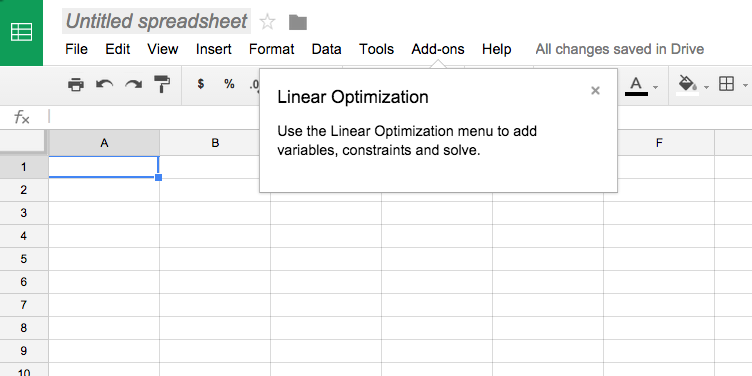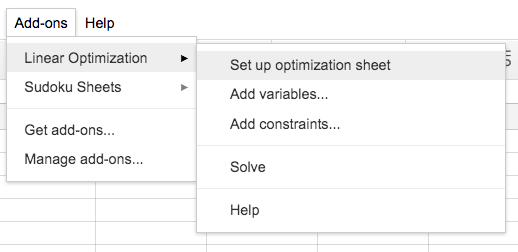After you select "Set up optimization sheet", your sheet will be customized for linear optimization problems: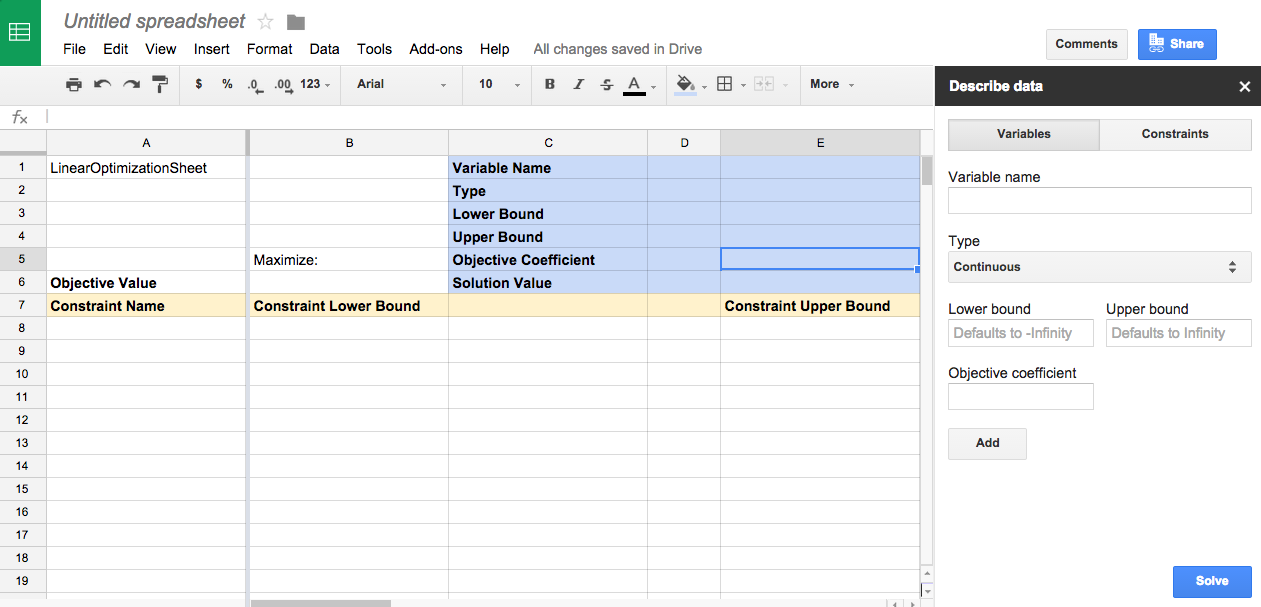Let's use the add-on to solve this simple system of linear equalities:

Maximize 3x + 4y subject to the following constraints:
 x + 2y ≤ 14 3x – y ≥ 0 x – y ≤ 2

Let's start by adding our objective function: 3x + 4y. On the right-hand panel, variables is already selected, so we'll add our first variable, with its objective coefficient of 3: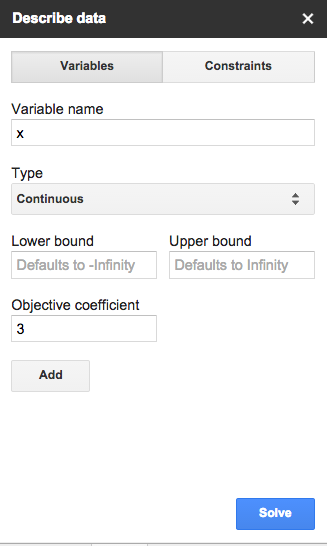That will populate the spreadsheet like so: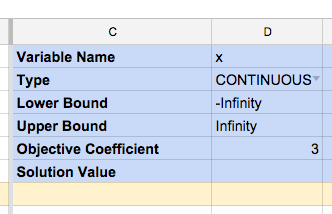We'll do the same for our 4y: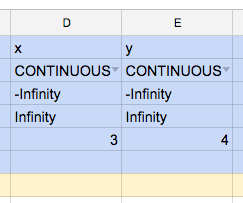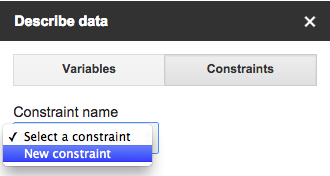Our first constraint, x + 2y ≤ 14, has no minimum value and a maximum value of 14. It doesn't matter much what we name it, so we'll call it "Swords":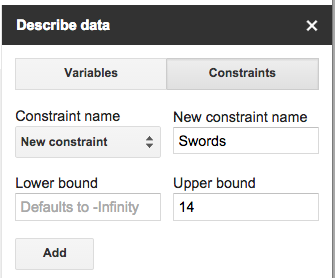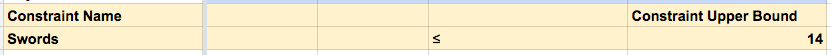Next we need to specify the coefficients for x and y. Since our constraint is x + 2y ≤ 14, those coefficients are 1 and 2 respectively. We can now select "Swords" as a constraint again to let us specify those coefficients, or we can add them directly to the spreadsheet.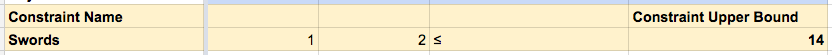Then we'll do the same for our other two constraints. The infinity and -infinity boundaries will be auto-populated; no need to type them.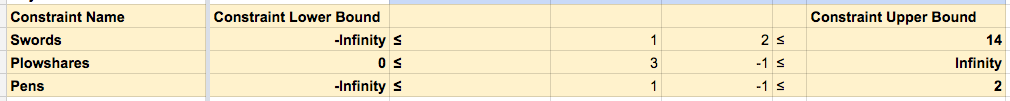Now we're ready to solve, which we can do either through the blue button in the "Describe data" panel, or through the Add-ons menu: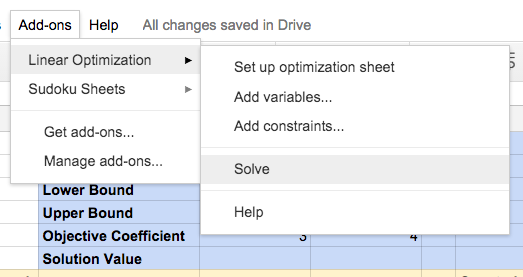The solution is filled in: with x=6 and y=4, the objective function is maximized at 34: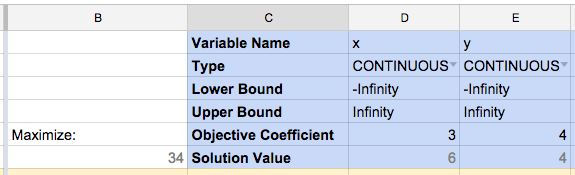The Linear Optimization add-on is useful for serious problems as well. The Stigler diet problem can be solved with a medium-sized Google Sheets file. Much larger problems can be solved as well; there's no practical limit on the number of variables or constraints, so long as it takes Google servers no longer than two minutes to solve the problem.

## How it works

The add-on is made possible by two underlying Google services. The first is Apps Script, which allows Google Sheets to be controlled programmatically. That's how the cells in the spreadsheet can be written to and read from.

The second service is the Linear Optimization Service for Apps Script, which allows programs to treat our linear solver as just another function. If the problems has any integer constraints, it's solved using SCIP; otherwise, it's solved using Glop.

The API has a timeout of five minutes, rather than the add-on's two minutes, so it's better-suited for larger optimization problems.